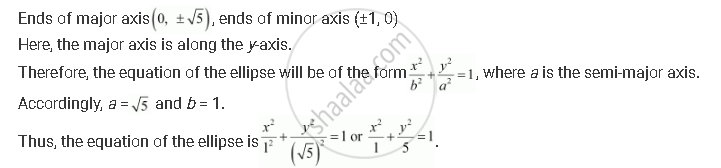CBSE (Arts) Class 11CBSE
Share
Notifications

View all notifications
Books Shortlist
Your shortlist is empty

# Find the Equation for the Ellipse that Satisfies the Given Conditions: Ends of Major Axis (0, +- Sqrt5), Ends of Minor Axis (±1, 0) - CBSE (Arts) Class 11 - Mathematics

Login
Create free account

Forgot password?
ConceptEllipse Standard Equations of an Ellipse

#### Question

Find the equation for the ellipse that satisfies the given conditions: Ends of major axis (0, +- sqrt5), ends of minor axis (±1, 0)

#### SolutionIs there an error in this question or solution?

#### APPEARS IN

Solution Find the Equation for the Ellipse that Satisfies the Given Conditions: Ends of Major Axis (0, +- Sqrt5), Ends of Minor Axis (±1, 0) Concept: Ellipse - Standard Equations of an Ellipse.
S